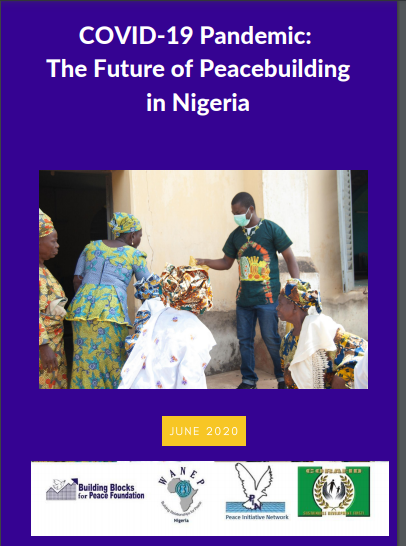# COVID-19 Pandemic: The Future of Peacebuilding in NigeriaEditions:PDF

Gl o b a l l y , C O V I D - 1 9 h a s u p e n d e d l i v e s , l i v e l i h o o d , e c o n o m i e s a n d c i v i l i z a t i o n s u s h e r i n g u s i n t o u n c h a r t e d t e r r i t o r y a n d u n c e r t a i n t i m e s i n j u s t o v e r f i v e m o n t h s . T h e p a n d e m i c h a s w i d e n e d i n e q u a l i t i e s , b r o u g h t u p n e w c o n f l i c t s a n d s h a k e n t h e t r u s t o f g l o b a l e c o n o m y a n d g o v e r n a n c e . I n A f r i c a , t h e s i t u a t i o n i s e v e n d i r e r .
F r a g i l e h e a l t h c a r e s y s t e m s , d e p e n d e n t e c o n o m i e s a n d w e a k g o v e r n m e n t i n s t i t u t i o n s h a v e l e d t o w o r s e n i n g c o n s e q u e n c e s f o r t h e c o n t i n e n t .

A l t h o u g h A f r i c a h a s r e c o r d e d c o m p a r a t i v e l y f e w e r o f f i c i a l c a s u a l t i e s t h a n o t h e r r e g i o n s , t h e i m p a c t c o u l d b e d e a d l i e r w i t h o n - g o i n g p o l i t i c a l c r i s e s a n d t h e l i k e l i h o o d o f f u r t h e r d e p r i v a t i o n o f t h e v u l n e r a b l e p o p u l a t i o n .

T h e r e f o r e , t h i s r e p o r t c o u l d n o t h a v e c o m e a t a b e t t e r t i m e t h a n n o w t h a t p e a c e b u i l d e r s a r e f a c e d w i t h r e s o l v i n g p r e - C O V I D - 1 9 c o n f l i c t a n d a l s o t h e n e w o n e s c e r t a i n t o a r i s e f r o m h u m a n r i g h t s a b u s e s , s h r i n k i n g c i v i c s p a c e , c o r r u p t i o n a n d e c o n o m i c h a r d s h i p .

Published:
Excerpt:

Globally, COVID-19 has brought disruptions and the creation of a "new normal. The pandemic has widened inequalities, brought up new conflicts and shaken the trust of global economy and governance. In Nigeria, the pandemic has exposed her weak health care system, destroyed means of livelihoods, dragged more people below the poverty line and led to gross human rights abuses. While gains in conflict-ravaged states like Borno, Yobe and Adamawa could be easily lost. Peacebuilders must step in to help but adapt to a socially distant future that is heavily dependent on virtual interactions.